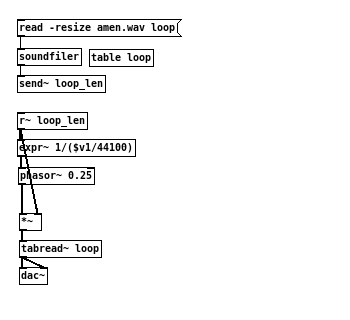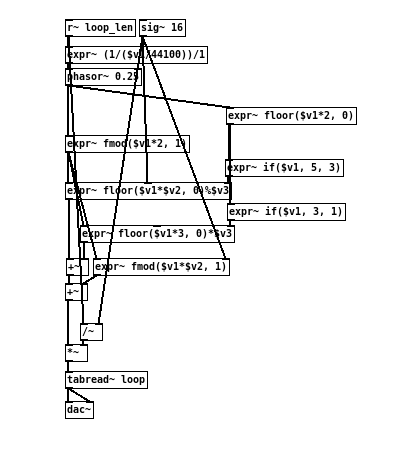```                  ______
/      |       ________
/       |____  /        \
/        |    \/   _      \
\       ___       /_\      \
\      \_/          .      \
\          /\_____/       /
\________/      |       /
|______/

```

# [THE_EXPR_METHOD] 011 Playing samples

20160525

Although the examples so far all uses simple sound synthesis to demonstrate the underlying principles of the signal-only methodology, they can easily be extended to control and manipulate audio samples as well.

As sound files are in essence arrays of values stored on disk, playing back an sample can be done simply by using a [phasor~] to systematically step through each of the values in the file.

To begin with, one must know the length of the chosen file, in number of samples. This value can be obtained from the output of the [soundfiler] object, and can then be multiplied with a sawtooth signal. The result is a complete indexing from the beginning to the end of the file, which the [tabread~] object can accept as input.

To resolve the “correct” rate for playback, however, can be somewhat ambiguous. On the one hand, there is the actual speed in which the sample was first recorded. On the other hand, there is the desired speed in which the current composition demands.

Assuming the goal is to playback at the recorded speed, the original sample rate also must be known. By dividing the length of the sound file with the sample rate, the time duration is therefore known, and can easily be converted into frequency.

The following example shows an simple playback setupSuch a result admittedly is not very interesting compositionally. However, by applying the principles that had been introduced previously, step counting for instance, one can easily make the necessary changes to give much more flexibility and possibilities.

This second example demonstrates the ability to slice the sample into definable sections, and rearrange them according to a modulated counter.Get the source here

Note that there is no amplitude envelope in the above example to correct the hard clipping at the edges of each sample sections. To achieve this, one can produce an signal in the shape of (isosceles) trapezoid signal to modulate over the sample values.

Exercise:

• Besides the frequency, what other parameters can be modified/modulated for sample playback?
• Are there other interesting counting method to experiment with?